StatLect

# Cross-covariance matrix

The cross-covariance matrix between two random vectorsandis a matrix containing the covariances between all the possible couples of random variables formed by one entry ofand one entry of.## Definition

This is a formal definition.

Definition Letbe arandom vector andbe arandom vector. The cross-covariance matrix betweenandis amatrix, denoted byand defined as follows: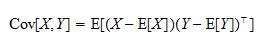Note that in the formula aboveis a column vector andis a row vector.

## Example

Define two random vectorsandas follows:The cross-covariance matrix betweenandis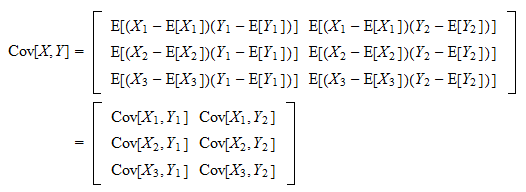## Special case

When, then the cross-covariance matrix coincides with the covariance matrix of: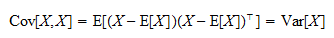## Autocovariance matrix

Letbe a sequence of random vectors, whereis a time-index.

Letbe a time lag. Then, the cross-covariance matrixis called autocovariance matrix.

## Lack of symmetry

Note that, in general, the cross-covariance is not symmetric.

In fact,and, in general,For example, ifisandis,isandis.

## Other properties

We have just demonstrated thatOther useful properties that can be easily derived from the definition (exercise!) are:

• formula in terms of cross-moments:• additivity: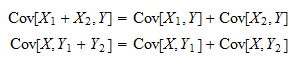• homogeneity:whereis a constant scalar;

• formula for linear transformations:whereandare constant vectors andandare constant conformable matrices.

## Use in time-series analysis

The cross-covariance matrix is often used in time-series analysis and in the theory of stochastic processes.

For example, it is used to define the concept of covariance stationarity for random vectors.

A sequence of random vectorsis said to be covariance stationary if and only if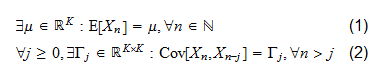whereandare integers.

Property (1) means that all the random vectors belonging to the sequencemust have the same mean.

Property (2) means that the cross-covariance between a term of the sequence () and the term that is locatedpositions before it () must always be the same, irrespective of howhas been chosen. In other words,depends only onand not on.

## Sum of covariance stationary vectors

Ifis a covariance stationary sequence of vectors, we can use the following important formula for the covariance matrix of a sum: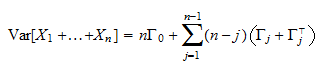Proof

This is demonstrated as follows: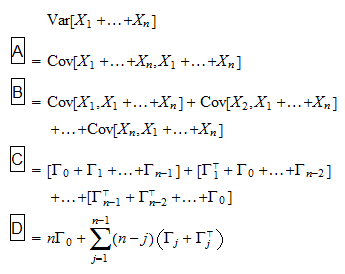where: in stepwe have used the fact that the cross-covariance of a vector with itself is equal to its covariance matrix; in stepwe have used the additivity property; in stepwe have used the stationarity of the sequence; in stepwe have re-grouped the summands.

## Use in asymptotic theory and statistical inference

Consider a sequence of covariance stationary random vectors.

The sample mean of the firstterms of the sequence isUnder some regularity conditions, the sample mean is asymptotically normal, with meanand asymptotic covariance matrix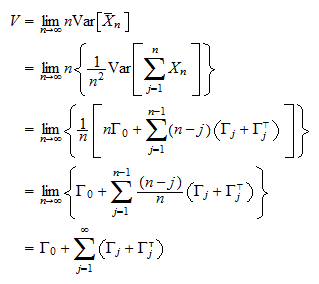where the cross-covariance matricesare defined as in equation (2) above.

In other words, if a multivariate central limit theorem for dependent sequences applies, thenwheredenotes convergence in distribution anddenotes a multivariate normal distribution with zero mean and long-run covariance matrix equal to.

## Cross-covariance function

Letbe a sequence of random vectors.

When the cross-covariance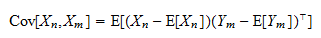is viewed as a function of the two indicesand, then it is called cross-covariance function.

Previous entry: Critical value

Next entry: Design matrix